Logistic regression — also known as logit regression, binary logit, or binary logistic regression — is a type of regression analysis used when the dependent variable is binary (i.e., has only two possible outcomes). It is used widely in many fields, particularly in medical and social science research.

Examples of situations where logistic regression can be applied are:

• Predicting the risk of developing heart disease given characteristics such as age, gender, body mass index, smoking habits, diet, and exercise frequency.
• Predicting whether a consumer will buy an SUV given their income, marital status, number of children, and how much time they spend outdoors.
• Predicting whether a student will pass an exam given their past grades, homework completion, and class attendance.

Logistic regression is a special case of a generalized linear model (GLM), which also includes linear regression, Poisson regression, and multinomial logistic regression.

## Theory

Linear regression is used to model a numeric variable as a linear combination of numeric independent variables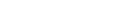weighted by the coefficients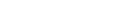: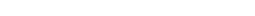Suppose instead that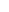is a binary variable. In the past, linear regression would also have been used. There are several disadvantages with this. These all stem from the fact that we are using a linear combination of numeric variables, which may be any number, to model a binary variable that has only two values.

The approach used by logistic regression is to model the log of the odds ratio of the outcomes instead: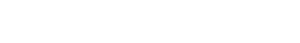where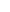is the probability of one of the two outcomes. The left-hand side is a function ofknown as the logit function, which has a range from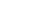to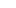:The closely related probit regression differs from logistic regression by replacing the logit function with the inverse normal cumulative distribution.

A logistic regression model is fit by estimating the coefficients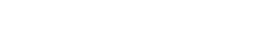using maximum likelihood estimation. This is because no closed-form solution exists, unlike for linear regression. In practice, logistic regression is carried out using statistical software. For example, in R, the glm function can be used (with the setting family = binomial(link = 'logit')).

## Output

The output typically consists of estimates of the coefficients, as well as their corresponding standard errors and Wald z-statistics. Using the z-statistics, the coefficients are tested for significance from zero using a z-test. A likelihood-ratio test may also be conducted. This will determine if the predictors provide a significantly improved model fit over a null model with no predictors. In addition, pseudo-R2s analogous to R2 from linear regression can be computed, such as the McFadden R2, to assess the goodness of fit of a logistic regression model.

Check out more Beginner's Guides, or head to the rest of our blog to read more!